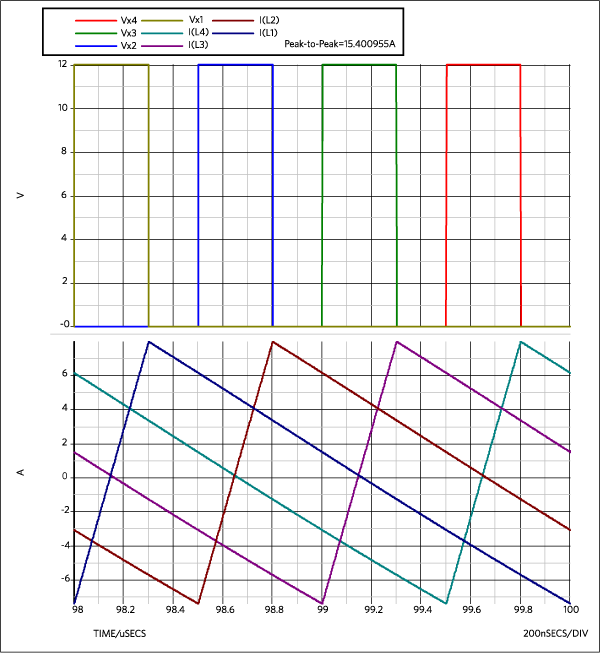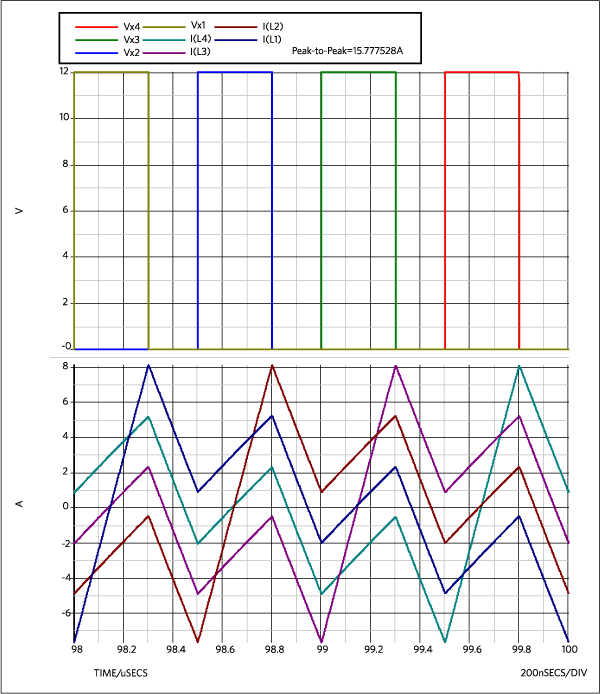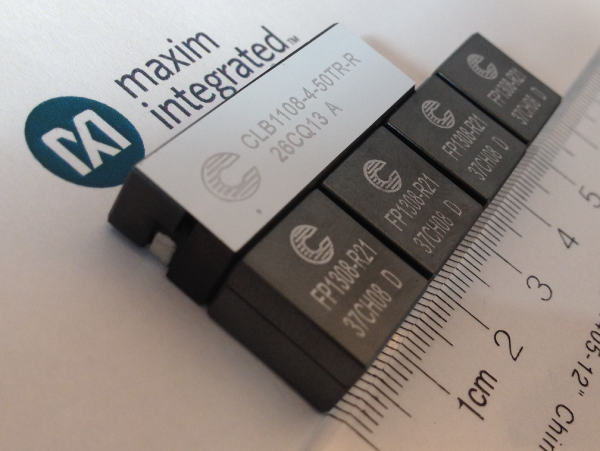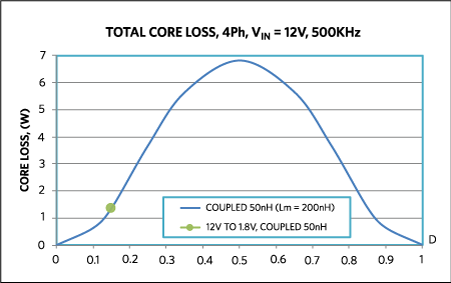# Addressing Core Loss in Coupled Inductors

### Abstract

Core loss in inductors can adversely affect system performance. Yet, predicting core loss is a complicated endeavor, especially in complex structures such as coupled inductors. This article examines core loss and the resulting effects that should be considered. The article also discusses how the core loss in coupled inductor design can be approached in order to provide a complete power delivery solution.

### Introduction

Magnetic components, such as inductors and transformers, are often an important part of power conversion. But core loss in these magnetic components can affect system performance significantly, starting with efficiency. Magnetic components can limit a choice for the switching frequency and greatly influence the overall solution size. Core loss is generally a complicated area of research 1-2, 12, with parametric description of how the losses depend on different parameters. When coupled inductors were introduced and implemented in many commercial products to derive substantial system benefits 3-9, core loss estimates became even more complex. The difficulties of core loss prediction for coupled inductors are generally associated with many different core cross sections, several different current waveforms that interact magnetically, and the different directions for many fluxes in the core: coupling, and leakage fluxes.

This article provides some details of core losses in coupled inductors and necessary effects to consider. It also illustrates that the design of the coupled inductors is more complex than discrete inductor design, which has a single magnetic flux and uniform cross section for it. This complexity highlights the significance of the developed coupled inductor parts available from licensed suppliers, as a lot of effort and validation must happen for each new design.

### Basic Core Loss Equation

The basic core loss is a famed Steinmetz equation (1), where B is the peak flux density, f is the frequency of applied sinusoid, Pv is the time-average power loss per unit volume, and k, a, ß are material parameters. These parameters are referred to as Steinmetz parameters, and are found by fitting the measured data for a particular material. The original equation from Steinmetz (proposed in 1892) did not have a dependence on the frequency of the sine wave excitation, which was added later.

 PV = k × ƒa × Bß (Eq. 1)

This is a basic core loss equation, which offers no physical meaning but rather a parametric fit to the measured data; therefore, a core loss can be predicted in some region of conditions around where initial measurements were conducted. This equation is in many ways not very accurate, as it works only for sinusoidal waveforms and in particular conditions. Many switching converters have square wave voltages applied to the magnetics, which usually results in triangular ripple waveforms for the current. This certainly affects the magnetic flux and related core losses. What also is a big problem is the fact that the fit parameters k, a, and ß severely depend on different conditions, such as temperature, DC bias, and frequency.

A very good overview of the historical improvements for core loss modeling was presented at APEC 2012 1. A popular equation often used in the present industry relates to an improved generalized Steinmetz equation (iGSE) 2. The general equation for iGSE is shown as (2), where ki is expressed as (3). Integrating over time would provide the actual (averaged) core loss (4).

This is the content for Layout P Tag(Eq. 2)(Eq. 3)(Eq. 4)

While iGSE introduces a big improvement for core loss estimation for non-sinusoidal waveforms, other effects would still have to be considered on top of it, such as dependence of the fit parameters on temperature, DC bias, and frequency. Practically, as magnetic flux density relates to the current in the winding of the inductor, it is easy to see in (4) that changes in current waveforms would be a good indication of the core loss change. For particular core and winding geometry, as well as particular switching circuit, current ripple can be calculated and translated into magnetic flux density in the core.

Typical discrete inductor has a single winding. For high-current, low-voltage applications it is often single turn or staple. The related core often has a simple shape and a single magnetic flux path, wrapping around the single turn winding. It is, therefore, relatively straightforward to define the flux density in that single flux path and relate it to the current in the winding. Then the core loss can be estimated for that single magnetic flux.

### Impact of Coupled Inductors on System Performance

When they were introduced, coupled inductors represented a significant advance in system performance for multiphase converters 3-9. Different designs have been developed over the years, with different geometries and different numbers of coupled phases. In terms of the core loss estimates, such complicated construction of the magnetics represents significant challenges.

The peak-to-peak current ripple in a traditional uncoupled buck converter can be expressed as a relatively simple equation (5), where Vin is input voltage, Vo is output voltage, L is inductance value, D is a duty cycle (D = Vo/Vin for a buck converter), and fS is a switching frequency.(Eq. 5)

When the inductor windings are coupled in a multiphase converter, a simple equation (5) for the current ripple is modified into (6), where ? = Lm/Lk is a coupling coefficient (Lm is magnetizing or mutual inductance, Lk is a leakage inductance) and Nph is a number of coupled phases 8.(Eq. 6)

The figure of merit (FOM) in (6) for a whole range of the duty cycle can be derived from equations in 9, using familiar and more convenient parameters, as (7).(Eq. 7)

This FOM expression (7) is valid for a particularregion of the duty cycle D, where index k changes in 0 < k < (Nph–1) range.

Figure 1 illustrates the current ripple waveforms in a four-phase buck converter for discrete 200nH inductors, Vin = 12V, Vo = 1.8V, and fS = 500kHz. Figure 2 shows the same waveforms but for a 50nH coupled inductor. The choice of 50nH coupled and 200nH discrete inductor values is clear in Figure 3: the current ripple in these inductors is similar in the 12V to 1.8V application (D = 0.15). The same peak-to-peak ripple would ensure the same RMS in all circuit waveforms, as well as the same switching losses, implying similar efficiency expectations. The benefit of a coupled inductor in this case is that the same system efficiency can be achieved with four times smaller inductance value for the transient, which implies smaller total magnetics size, as well as dramatically smaller output capacitance.

As shown in Figure 1 and Figure 2, 200nH discrete and 50nH coupled inductors do produce similar peak-to-peak current ripple in the conditions of the application specified above.Looking at current ripple for a coupled inductor in Figure 3, one can assume that the flux density would relate to the curve of the plotted current ripple, which would in turn affect the core loss in (2). Depending on the actual Steinmetz parameters for the core material in particular conditions, one can then expect that the core loss would follow a shape similar to the current ripple curve from Figure 3, modified by some degree function from (2).

However, this would not be a correct assumption.

To illustrate why the core loss plot of the coupled inductor does not really correspond to the shape of current ripple in one phase with related local minimums (Figure 3), the curve of current difference between the first phase and the three others are plotted in Figure 5. The actual current in phase 1 (IL1) is plotted as a reference for two switching periods, and then the difference curves are added: IL1–IL2, IL1–IL3, and IL1–IL4.

If the currents in all phases were forced to be equal at the same time, for example all as IL1 in Figure 2 or Figure 4, then the mutual flux of inversely coupled windings would be exactly zero. The magnetic flux would then be only in the leakages (independent fluxes in each winding), and the total core loss would actually correspond to the peak-to-peak current ripple amplitude in a single phase. As a result, the core loss curve would show similar local minimums as the current ripple curve for coupled inductor in Figure 3. But, clearly, the current waveforms in a real circuit are not equal in different phases, so the fluxes are also present in the paths for mutual inductances between the phases. These fluxes, and related core loss, relate to the current difference between the phases, not the particular current ripple amplitude itself. The trapezoidal waveform shape is yet another departure from the Steinmetz assumption, which requires attention to other magnetic memory effects not captured in typical loss models.

To further complicate the issue, notice that while the current difference curves IL1–IL2 and IL1–IL4 in Figure 5 are on top of each other and the second phase IL2 is next to the base phase IL1, the IL4 phase is actually located on the opposite side of the stretched magnetic core. This would imply that the core loss would be larger for the flux between the first and fourth phases than between the first and second, as it would have to travel a much larger distance in the ferrite.

Another consideration is that the leakage flux is also traveling in a different path than the coupling fluxes. For the coupled inductor design in Figure 6, the leakage flux goes into a plate right on top of the windings, returning in a very short vertical loop, while the coupling fluxes run in horizontal loops around the main core between the windings. The leakage flux and its core loss contribution would clearly relate to the actual current waveform of the particular winding, so some relation of that part of the core loss to the local minimums of current ripple curve in Figure 3 is expected. However, it is inadequate to assume that linear superposition will apply because the core material loss scales with an exponential relationship, based on our Steinmetz assumption, requiring a designer to compute the total flux in each of these paths. The flux distribution implies that the physical difference of leakage and coupling flux paths can affect their relative impact on the total. In other words, building a coupled inductor with an excessively long (and also too narrow) path for the leakage fluxes would distort the total core loss curve towards more pronounced local minimums of the current ripple in Figure 3.Simulating Core Loss in Four-Phase Coupled Inductor

Maxwell 3D software performed simulation for the core loss for the four-phase coupled inductor. The off-the-shelf coupled inductor CLB1108–4–50TR was modeled 10, and actual parts are shown in Figure 6. The following Steinmetz parameters for 3F4 ferrite were used in core loss simulation (8):(Eq. 8)

The results for core loss are plotted in Figure 7. It is clear that the core loss curve does not correspond to the shape of the current ripple curve for the coupled inductor in Figure 3. It does not have the minimum points that would correspond to the points of local minimum current ripple (D = 0.25, D = 0.5, D = 0.75). This implies that the main loss contribution is coming from the coupling fluxes between the windings. Such a conclusion makes sense, as leakage fluxes are typically designed to be several times smaller than the coupling fluxes (Lm/Lk > 1) to achieve a benefit of significant current ripple cancellation. Since the core loss depends on flux density in a very non-linear fashion (see example values (8) to substitute into (1)), several times lower flux should generally relate to significantly smaller core loss. In addition, the actual design of the investigated coupled inductor in Figure 6 has a very short and wide path for the leakage flux on top of each winding, while coupling fluxes pretty much fill the full length of the main core, going between different windings. Longer flux path would generally associate with a larger volume that generates the core loss.The implications of this study are that coupled inductor designs add further complications for core loss evaluations. Not only does the peak-to-peak current ripple in each phase matter, but also the difference between currents in different phases is an important consideration. For a relative simple core structure with a low number of phases, engineers can still estimate this problem analytically by establishing equivalent circuits for common mode current and differential mode current between phases 12, or by simply calculating the magnetic flux in the core according to differences in phase current. However, for designs with a higher number of phases and more complex core structure, magnetic flux density could be very different in different core sections. Building an analytical model would require dividing the core analysis into many finer sections based on the actual core shape, which is starting to approach a “manual” finite-element analysis. Since most of the coupled inductor would be a customized design based on the specific applications, the analytical model would not be universal and needs to be customized for each design. Letting designers build models for each design would be very inefficient on both time and cost. Computer-aided finite-element analysis (FEA) would make more sense here.

One thing worth noting: for coupled inductor analysis, engineers should pay special attention to the choice of Steinmetz parameters. As we mentioned before, Steinmetz parameters are purely empirical, which means for different operating ranges (frequency and flux density), engineers can choose different Steinmetz parameters to get more accurate estimates. For coupled inductor design, Steinmetz parameters would need to be chosen for the most relevant conditions, which typically implies evaluating the core materials in house as typical frequency of the magnetic flux fundamental is switching frequency multiplied by the number of coupled phases (see Figure 3). Such multiplied frequency is typically much higher than core loss information available from vendors. For example, fS = 500kHz per phase application condition for core loss in Figure 7 actually implies that the needed Steinmetz parameters would need to be correct at 500kHz x 4 = 2MHz and harmonics above it. However, if the circuit needs to operate by just firing one or two phases in some circumstances, the Steinmetz parameter choice would apparently be different. Furthermore, for some extreme core structure design, currents from different phases, magnetizing current, and leakage current may apply orthogonal flux in certain core sections, which will further complicate the Steinmetz parameter choice and core loss evaluation.

### A Complete Power Delivery Solution

Coupled inductor design is challenging for inductor vendors. To provide a complete power delivery solution, optimization must happen in all key areas, including the power stage, control, magnetics, and output capacitance. The solution’s efficiency, transient performance, size, and cost can better meet particular customer priorities for the application. Core loss would be a part of such optimization. This is why typical coupled inductor design usually is proposed by a power solution manufacturer first, optimized together with the other components of the solution. Then, with feedback from the magnetics vendor and some practical iterations and tests, the magnetics design can be approved for manufacturing.

By examining this core loss discussion, considering these equations, and using FEA tools (such as ANSYS Maxwell), designers can find solutions for power optimization, as there is no straightforward way to gain a core loss estimate in a coupled inductor design with reasonable accuracy in a simple analytical form.

### 参考电路

1. Charles R. Sullivan, “Overview of core loss prediction (and measurement techniques) for non-sinusoidal waveforms,” presented in a seminar at IEEE Applied Power Electronics Conference and Exposition, APEC 2012. http://engineering.dartmouth.edu/inductor/Sullivan_APEC_2012_core_loss%20overview_with_references.pdf

2. K. Venkatachalam, C. R. Sullivan, T. Abdallah, and H. Tacca, "Accurate prediction of ferrite coread620 loss with nonsinusoidal waveforms using only Steinmetz parameters" IEEE Workshop on Computers in Power Electronics (COMPEL), 2002. http://inductor.thayerschool.org/index.shtml

3. Pit-Leong Wong, Peng Xu, Bo Yang, and Fred C. Lee, "Performance improvements of interleaving VRMs with coupling inductors," IEEE Trans. on Power Electronics, vol. 16, no. 4, pp. 499–507, 2001.

4. Aaron M. Schultz and Charles R. Sullivan, "Voltage converter with coupled inductive windings, and associated methods," U.S. Patent 6,362,986, March 26, 2002.

5. Jieli Li and Charles R. Sullivan, Angel Gentchev, “Method for making magnetic components with N-phase coupling, and related inductor structures,” U.S. Patent 7,352,269, April 1, 2008.

6. Jieli Li, Charles R. Sullivan, Aaron Schultz, “Coupled inductor design optimization for fast-response low-voltage DC-DC converters,” in Proceedings of IEEE Applied Power Electronics Conference and Exposition, APEC 2002, pp. 817–823 vol.2.

7. Peng Xu, Jia Wei, Kaiwei Yao, Yu Meng, Fred C. Lee, “Investigation of candidate topologies for 12V VRM,” in Proceedings of IEEE Applied Power Electronics Conference and Exposition, APEC 2002, pp. 686-692 vol.2.

8. Jieli Li, Anthony Stratakos, Charles R. Sullivan, Aaron Schultz, “Using coupled inductors to enhance transient performance of multi-phase buck converters,” in Proceedings of IEEE Applied Power Electronics Conference and Exposition, APEC 2004, pp. 1289–1293 vol.2.

9. Yan Dong, “Investigation of Multiphase Coupled-Inductor Buck Converters in Point-of-Load Applications,” Virginia Tech, PhD Thesis 2009.

10. T. Schmid, A. Ikriannikov, “Magnetically Coupled Buck Converters,” in Proceedings of IEEE Energy Conversion Congress and Exposition, ECCE 2013, pp. 4948-4954.

11. Data sheet for CLB1108-4-50TR-R (four-phase 50nH coupled inductor)

12. David O. Boillat, Johann W. Kolar, “Modeling and experimental analysis of a coupling inductor employed in a high performance AC power source,” Proceedings of the International Conference on Renewable Energy Research and Applications (ICRERA 2012), Nagasaki, Japan, November 11-14, 2012. A similar version of this application note appeared in December 2016, in EDN Network.